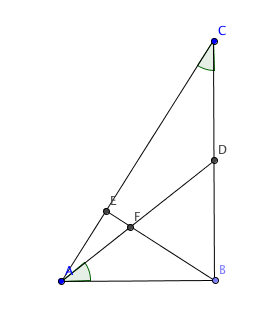# Triangle with an Altitude

Geometry Level 4

$ABC$ is a right triangle with $\angle ABC = 90^\circ$. Point $D$ is the midpoint of $BC$, and point $E$ is the foot of the perpendicular from $B$ to $AC$. $AD$ and $BE$ intersect at $F$. If $AB = 36$ and $\angle BAD = \angle BCA$, what is the value of $FE^2$?×